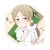International Conference on Algorithmic Learning Theory（ALT）是由算法学习理论协会（AALT），和其他相关活动一起来推广学习理论。官网链接：http://alt2019.algorithmiclearningtheory.org/0+0+0+0+0+0+0+0+0+0+0+0+0+0+0+0+0+0+0+0+0+0+0+0+0+0+0+0+0+0+0+0+0+0+0+0+0+0+0+0+0+0+0+0+0+
Top